#install.packages("psych")
#install.packages("gpairs")
#install.packages("gplots")
#install.packages("coefplot")
#install.packages("car")
#install.packages("corrplot")
#install.packages("alr3")
#install.packaged("MASS")
#install.packages("mlogit")
library(MASS)
library(alr3)
require(car)
require("psych")
require(gpairs)
require(corrplot)
require(gplots)
require(coefplot)


setwd("C:\\Users\\armop\\Dropbox\\PHD\\Teaching\\AGBU505\\2019\\Lecture1") ##Start by defining directory


After loading the data start the usual procedure to check the correctness of the loaded data. Check whether the loaded data has correct dimensions, print the first and last few observations.

dim(df)


<ol class=list-inline> <li>500</li> <li>8</li> </ol>

head(df)

<th scope=col>weekend</th><th scope=col>num.child</th><th scope=col>distance</th><th scope=col>rides</th><th scope=col>games</th><th scope=col>wait</th><th scope=col>clean</th><th scope=col>overall</th>
yes 0 114.6482682 68 64 86 49
yes 2 27.0141082 73 80 84 68
no 1 63.3009879 75 74 86 62
yes 0 25.9099382 68 69 87 38
no 4 54.7183178 83 78 84 69
no 5 22.6793476 75 51 77 29
tail(df)

<th scope=col>weekend</th><th scope=col>num.child</th><th scope=col>distance</th><th scope=col>rides</th><th scope=col>games</th><th scope=col>wait</th><th scope=col>clean</th><th scope=col>overall</th><th scope=row>495</th><th scope=row>496</th><th scope=row>497</th><th scope=row>498</th><th scope=row>499</th><th scope=row>500</th>
no 5 41.4701078 80 80 87 57
no 0 11.0525885 67 72 87 48
yes 0 8.1877486 79 85 89 48
no 2 45.1774090 88 89 91 74
no 3 27.0883878 79 84 86 57
no 1 38.4087681 84 81 83 65

Check for NAN or missing values

sum(is.na(df)) #Check for NAN or missing values


0

It is good idea to summerize your data and investigate it.

describe(df)

<th scope=col>vars</th><th scope=col>n</th><th scope=col>mean</th><th scope=col>sd</th><th scope=col>median</th><th scope=col>trimmed</th><th scope=col>mad</th><th scope=col>min</th><th scope=col>max</th><th scope=col>range</th><th scope=col>skew</th><th scope=col>kurtosis</th><th scope=col>se</th><th scope=row>weekend*</th><th scope=row>num.child</th><th scope=row>distance</th><th scope=row>rides</th><th scope=row>games</th><th scope=row>wait</th><th scope=row>clean</th><th scope=row>overall</th>
1 500 1.48200 0.5001763 1.00000 1.47750 0.00000 1.0000000 2.0000 1.0000 0.071830669-1.9988260 0.02236857
2 500 1.73800 1.4959384 2.00000 1.61000 1.48260 0.0000000 5.0000 5.0000 0.437142150-0.7509303 0.06690040
3 500 31.04751 33.1454452 19.01909 24.64603 17.25512 0.5267228 239.1921 238.6654 2.574233780 8.9959900 1.48230937
4 500 80.38200 5.4150458 80.00000 80.35250 5.93040 67.0000000 94.0000 27.0000 0.060117097-0.4848875 0.24216821
5 500 74.14400 8.1249797 74.00000 74.20000 8.89560 52.0000000 96.0000 44.0000 -0.049533738-0.3451199 0.36336014
6 500 73.41600 10.7518439 74.00000 73.50500 11.86080 43.0000000 103.0000 60.0000 -0.075266072-0.2293318 0.48083708
7 500 85.39200 5.1333262 85.00000 85.38750 5.93040 72.0000000 98.0000 26.0000 0.008375348-0.4473279 0.22956933
8 500 52.87200 16.0763339 52.00000 52.51000 16.30860 6.0000000 102.0000 96.0000 0.196187378-0.1597183 0.71895551

or you can use different command

summary(df)

 weekend     num.child        distance            rides           games
no :259   Min.   :0.000   Min.   :  0.5267   Min.   :67.00   Min.   :52.00
yes:241   1st Qu.:0.000   1st Qu.: 10.3181   1st Qu.:77.00   1st Qu.:68.00
Median :2.000   Median : 19.0191   Median :80.00   Median :74.00
Mean   :1.738   Mean   : 31.0475   Mean   :80.38   Mean   :74.14
3rd Qu.:3.000   3rd Qu.: 39.5821   3rd Qu.:84.00   3rd Qu.:80.00
Max.   :5.000   Max.   :239.1921   Max.   :94.00   Max.   :96.00
wait            clean          overall
Min.   : 43.00   Min.   :72.00   Min.   :  6.00
1st Qu.: 65.75   1st Qu.:82.00   1st Qu.: 41.75
Median : 74.00   Median :85.00   Median : 52.00
Mean   : 73.42   Mean   :85.39   Mean   : 52.87
3rd Qu.: 81.00   3rd Qu.:89.00   3rd Qu.: 63.00
Max.   :103.00   Max.   :98.00   Max.   :102.00


For summerizing discrete data use function table()

table(df$weekend) #for discrete data   no yes 259 241  Before modeling, there are two important things to check: that each individual variable has a reasonable distribution, and that joint relationships among the variables are appropriate for modeling. Start by visualizing data. #Visualize the data pairs(formula = ∼ weekend + num.child + distance +rides + games + wait + clean + overall, data=df,main="")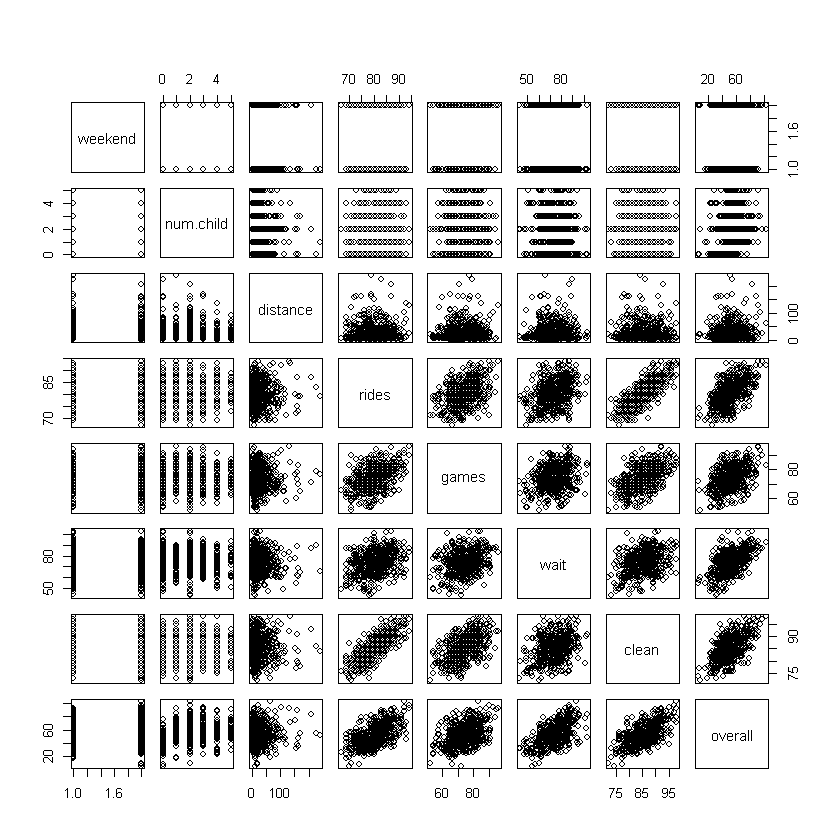gpairs(df)  Loading required package: grid Loading required package: lattice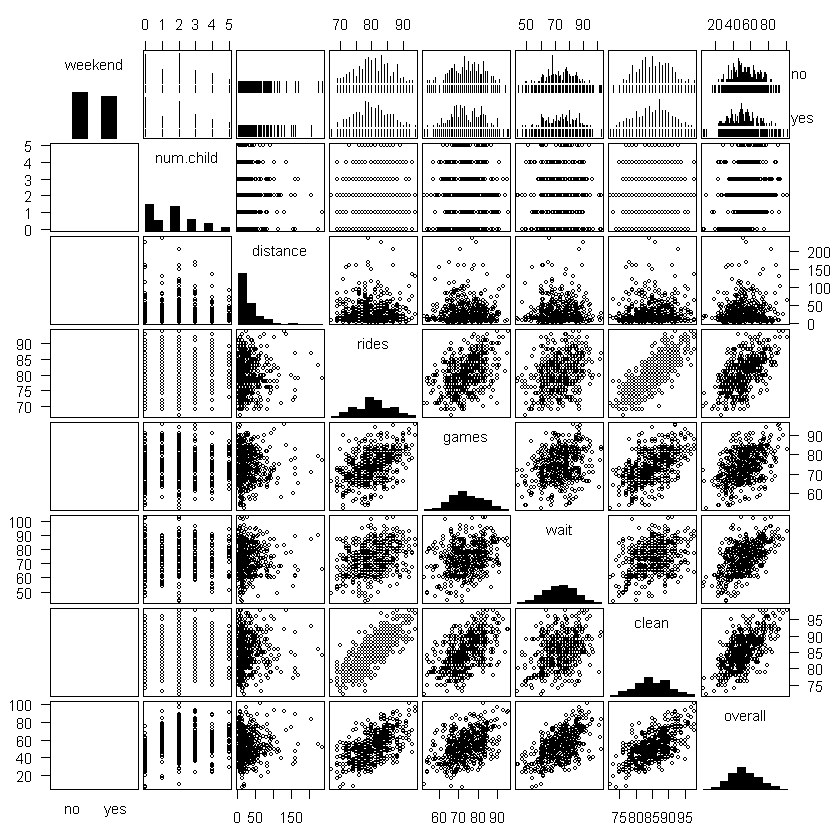The difference of the above two function is on how they handle the discrete values. To check the relationships among variables, we examine the bivariate scatterplots shown Figure above. They show few concerns apart from the need to transform distance. For example, the pairwise scatterplots of our continuous measures are generally elliptical in shape, which is a good indication that they are appropriate to use in a linear model. One question, however, concerns the fact that the variables in the lower right of figure are positively correlated. df$logdist=log(df$distance)  gpairs(df[,-3])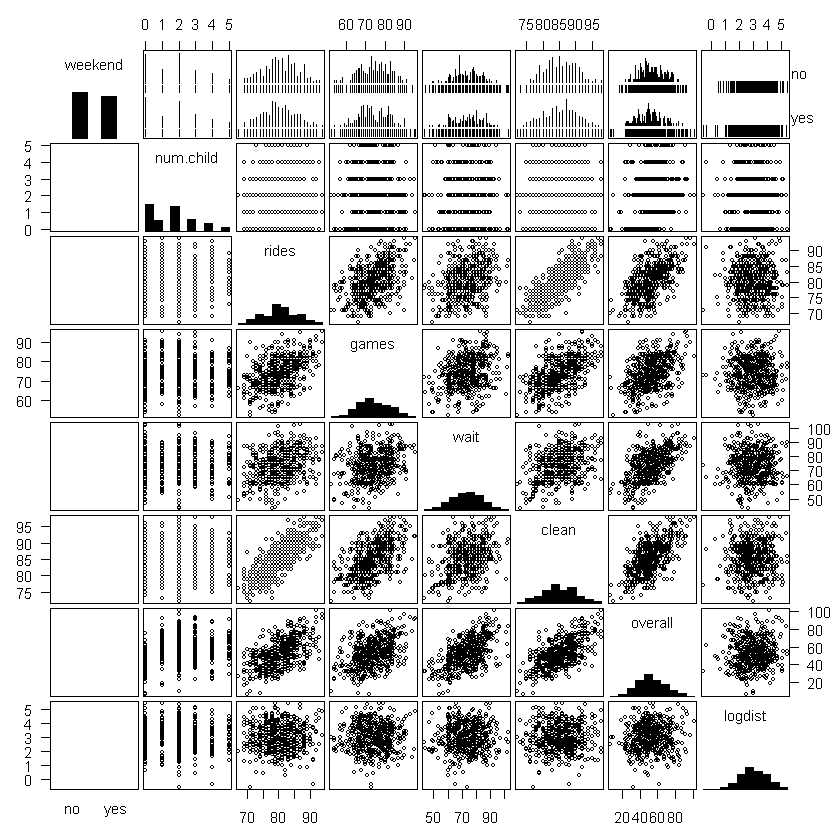head(df)  <th scope=col>weekend</th><th scope=col>num.child</th><th scope=col>distance</th><th scope=col>rides</th><th scope=col>games</th><th scope=col>wait</th><th scope=col>clean</th><th scope=col>overall</th><th scope=col>logdist</th> yes 0 114.6482682 68 64 86 49 4.741869 yes 2 27.0141082 73 80 84 68 3.296359 no 1 63.3009879 75 74 86 62 4.147901 yes 0 25.9099382 68 69 87 38 3.254626 no 4 54.7183178 83 78 84 69 4.002198 no 5 22.6793476 75 51 77 29 3.121454 Although scatterplots provide a lot of visual information, when there are more than a few variables, it can be helpful to assess the relationship between each pair with a single number. cor(df[,2:8])  <th scope=col>num.child</th><th scope=col>distance</th><th scope=col>rides</th><th scope=col>games</th><th scope=col>wait</th><th scope=col>clean</th><th scope=col>overall</th><th scope=row>num.child</th><th scope=row>distance</th><th scope=row>rides</th><th scope=row>games</th><th scope=row>wait</th><th scope=row>clean</th><th scope=row>overall</th> 1.00000000 -0.012136454-0.027697383 0.012343448-0.020994861-0.0079981400.34242021 -0.01213645 1.000000000-0.008598235-0.008036711-0.005848262 0.0029190320.08829766 -0.02769738 -0.008598235 1.000000000 0.453413358 0.315824769 0.7890047480.57772686 0.01234345 -0.008036711 0.453413358 1.000000000 0.299620997 0.5164607480.43325421 -0.02099486 -0.005848262 0.315824769 0.299620997 1.000000000 0.3668495040.56539527 -0.00799814 0.002919032 0.789004748 0.516460748 0.366849504 1.0000000000.62814674 0.34242021 0.088297655 0.577726856 0.433254206 0.565395275 0.6281467421.00000000 corrplot.mixed(corr=cor(df[ , 2:8], use="complete.obs"),upper="ellipse", tl.pos="lt")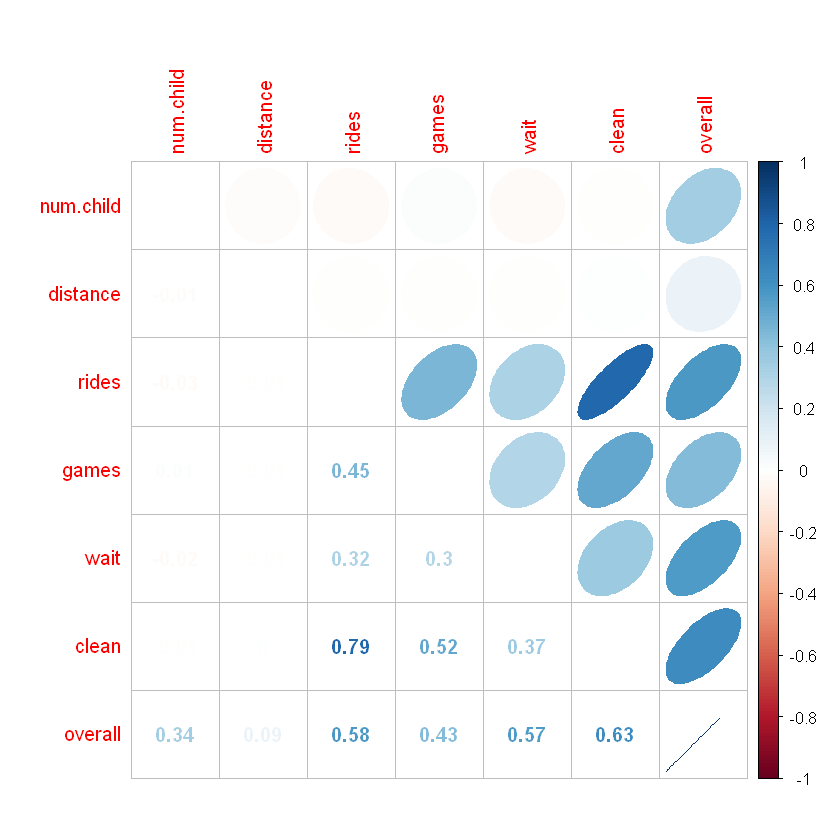A correlation plot produced using corrplot.mixed() from the corrplot package is an easy way to visualize all of the correlations in the data. Numeric values of r are shown in the lower triangle of the matrix. The upper triangle displays ellipses (because we used the argument upper=”ellipse”). These ellipses are tighter, progressively closer to being lines, for larger values of r, and are rounder, more like circles for r near zero. They are also shaded blue for positive direction, and red for negative (and show corresponding positive or negative slope). Correlation coefficient r measures the linear association between two variables. If the relationship between two variables is not linear, it would be misleading to interpret r. # Modeling Now we are ready for model selection and validation process. We start with the Linear Model with a Single Predictor. The goal of a satisfaction drivers analysis is to discover relationships between customers’ satisfaction with features of the service (or product) and their overall experience. For example, to what extent is satisfaction with the park’s rides related to overall experience? Is the relationship strong or weak? Like most other R functions, lm() returns an object that we can save and use for other purposes. Typically, we assign the result of lm() to an object that is used in subsequent lines of code. For example, we can assign the result of lm() to a new object m1: m1=lm(overall ∼ rides, data=df)  In the output, R repeats the model for reference and reports two coefficients, which are the intercept and the slope of the fitted line. Those can be used to determine the best estimate for any respondent’s report of overall based on knowing his or her value for rides. m1$coefficients


<dl class=dl-horizontal> <dt>(Intercept)</dt> <dd>-84.9968794504225</dd> <dt>rides</dt> <dd>1.71517105136004</dd> </dl>

typeof(m1$coefficients)  ‘double’ For example, from this model we would expect that a customer who gives a rating of 90 for satisfaction with rides would give an overall rating of: m1$coefficients+m1$coefficients*90  (Intercept): 69.3685151719808 plot(overall ∼ rides, data=df,xlab="Satisfaction with Rides", ylab="Overall Satisfaction") abline(m1, col="blue")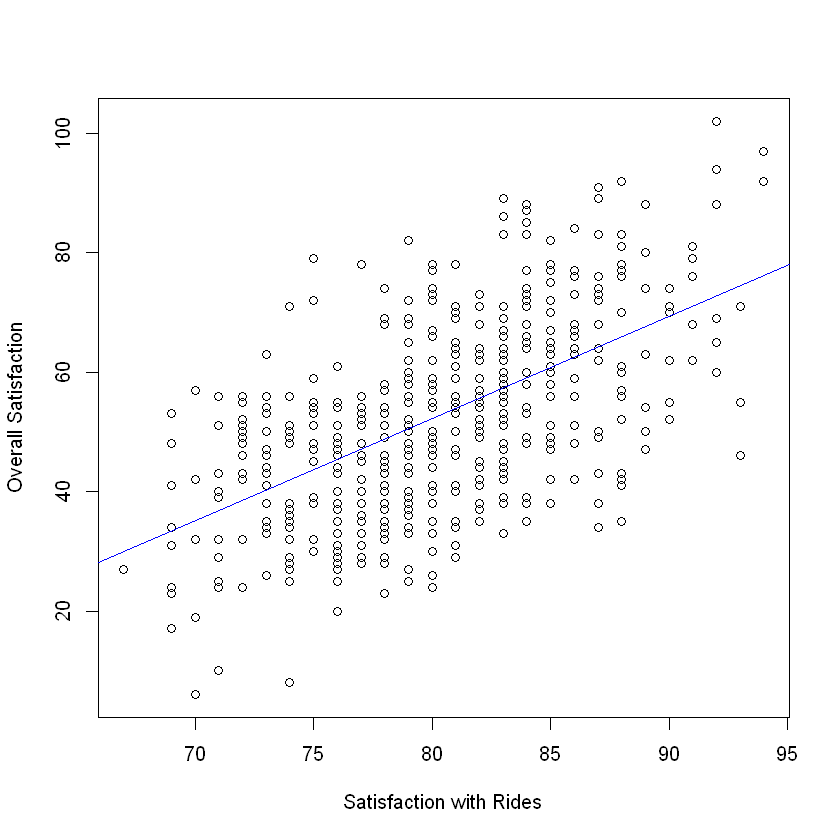The result is shown in abline() recognizes that it is dealing with an lm object and uses the slope and the intercept from m1 to draw the line. If you want more details about output summary(m1)  Call: lm(formula = overall ~ rides, data = df) Residuals: Min 1Q Median 3Q Max -33.926 -10.358 0.635 8.964 35.359 Coefficients: Estimate Std. Error t value Pr(>|t|) (Intercept) -84.9969 8.7483 -9.716 <2e-16 *** rides 1.7152 0.1086 15.795 <2e-16 *** --- Signif. codes: 0 '***' 0.001 '**' 0.01 '*' 0.05 '.' 0.1 ' ' 1 Residual standard error: 13.14 on 498 degrees of freedom Multiple R-squared: 0.3338, Adjusted R-squared: 0.3324 F-statistic: 249.5 on 1 and 498 DF, p-value: < 2.2e-16  t_value <- 1.7146/ 0.1085 print(t_value)   15.80276  p_value<-1-pt(t_value, df=498) print(p_value)   0  alpha=0.05 conf_int_l<-1.7146 -qt(alpha/2,498)*0.1085 conf_int_u<-1.7146 + qt(alpha/2,498)*0.1085 round(conf_int_l,3) round(conf_int_u,3)  1.928 1.501 round(confint(m1),3)  <th scope=col>2.5 %</th><th scope=col>97.5 %</th><th scope=row>(Intercept)</th><th scope=row>rides</th> -102.185-67.809 1.502 1.929 Cecking Model Validity and Model Fit par(mfrow=c(2,2)) plot(m1)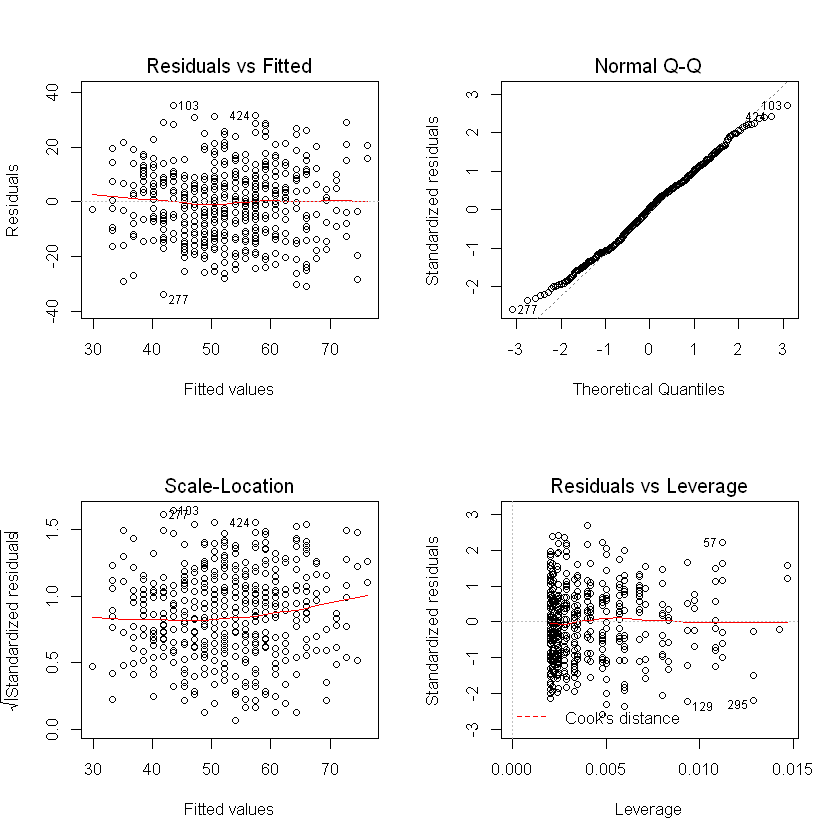1. The first plot (in the upper left corner) shows the fitted values versus residuals for$m1$. There is no obvious pattern between the fitted values for overall satisfaction and the residuals; this is consistent with the idea that the residuals are due to random error, and supports the notion that the model is adequate. 2. The second plot in the lower left of figure is similar to the first, except that instead of plotting the raw residual value, it plots the square root of the standardized residual. Again, there should be no clear pattern; if there were it might indicate a nonlinear relationship. Observations with high residuals are flagged as potential outliers, and R labels them with row numbers in case we wish to inspect them in the data frame. A common pattern in residual plots is a cone or funnel, where the range of errors gets progressively larger for larger fitted values. This is called heteroskedasticity and is a violation of linear model assumptions. Sometimes a transformation of the predictor or outcome variable will resolve heteroskedasticity. 3. The third result of plot() for lm objects is a Normal QQ plot. A QQ plot helps you see whether the residuals follow a normal distribution, another key assumption. It compares the values that residuals would be expected to take if they are normally distributed, versus their actual values. When the model is appropriate, these points are similar and fall close to a diagonal line; when the relationship between the variables is nonlinear or otherwise does not match the assumption, the points deviate from the diagonal line. In the present case, the QQ plot suggests that the data fits the assumption of the model. 4. The final plot in the lower right panel of figure again helps to identify potential outliers, observations that may come from a different distribution than the others. Outliers are a problem because, if they are far from other points, they unduly influence the fitted line. We do not want one or a very few observations to have a large effect on the coefficients. The lower right plot plots the leverage of each point, a measure of how much influence the point has on the model coefficients. When a point has a high residual and high leverage,bit indicates that the point has both a different pattern (residual) and undue influence (leverage). One measure of the leverage of a data point is Cook’s distance, an estimate of how much predicted (y) values would change if the model were re-estimated with that point eliminated from the data. If you have observations with high Cook’s distance, this chart would show dotted lines for the distances; in the present case, there are none. Still, in the lower right of figure, three points are automatically labeled with row numbers because they are potentially problematic outliers based on high standardized residual distance and leverage on the model. ## Dealing with Outliers I do not recommend routinely remove outliers yet I do recommend to inspect them and determine whether there is a problem with the data. Let inspect the identified points by selecting those rows: df[c(57, 129, 295),]  <th scope=col>weekend</th><th scope=col>num.child</th><th scope=col>distance</th><th scope=col>rides</th><th scope=col>games</th><th scope=col>wait</th><th scope=col>clean</th><th scope=col>overall</th><th scope=col>logdist</th><th scope=row>57</th><th scope=row>129</th><th scope=row>295</th> yes 2 63.2924892 83 93 98 102 4.147767 yes 0 11.8955070 72 54 74 6 2.476161 no 0 11.7447493 78 67 89 46 2.463406 In this case, none of the data points is obviously invalid (for instance, with values below 1 or greater than 100). Overall, figure looks good and suggests that the model relating overall satisfaction to satisfaction with rides is reasonable. Now that we’ve covered the basics of linear models using just one predictor, we turn to the problem of assessing multiple drivers of satisfaction. Our goal is to sort through all of the features of the park—rides, games, wait times, and cleanliness—to determine which ones are most closely related to overall satisfaction. ## Including Variables m2 <- lm(overall ∼ rides + games + wait + clean, data=df) summary(m2)  Call: lm(formula = overall ~ rides + games + wait + clean, data = df) Residuals: Min 1Q Median 3Q Max -32.101 -7.266 1.274 7.582 28.695 Coefficients: Estimate Std. Error t value Pr(>|t|) (Intercept) -125.16246 8.26476 -15.144 < 2e-16 *** rides 0.53948 0.14753 3.657 0.000283 *** games 0.16085 0.07115 2.261 0.024218 * wait 0.55497 0.04936 11.244 < 2e-16 *** clean 0.96028 0.16394 5.858 8.57e-09 *** --- Signif. codes: 0 '***' 0.001 '**' 0.01 '*' 0.05 '.' 0.1 ' ' 1 Residual standard error: 10.92 on 495 degrees of freedom Multiple R-squared: 0.5427, Adjusted R-squared: 0.539 F-statistic: 146.8 on 4 and 495 DF, p-value: < 2.2e-16  The R-squared increased to 0.5586, meaning that about half of the variation in overall ratings is explained by the ratings for specific features. The residual standard error is now 10.59, meaning that the predictions are more accurate. Our residuals also appear to be symmetric. par(mfrow=c(2,2)) plot(m2)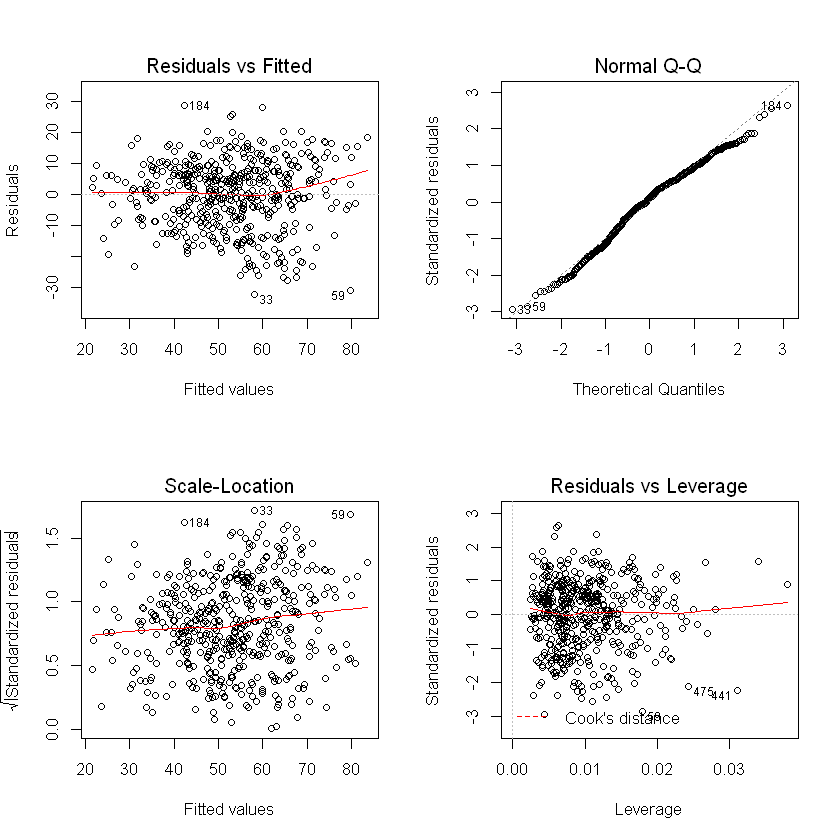Next we examine the model coefficients. Each coefficient represents the strength of the relationship between satisfaction with that feature and overall satisfaction, conditional on the values of the other predictors. All four features are identified as being statistically significant. coefplot(m2, intercept=FALSE, outerCI=1.96, lwdOuter=1.5, ylab="Rating of Feature",xlab="Association with Overall Satisfaction")  Warning message: "Ignoring unknown aesthetics: xmin, xmax"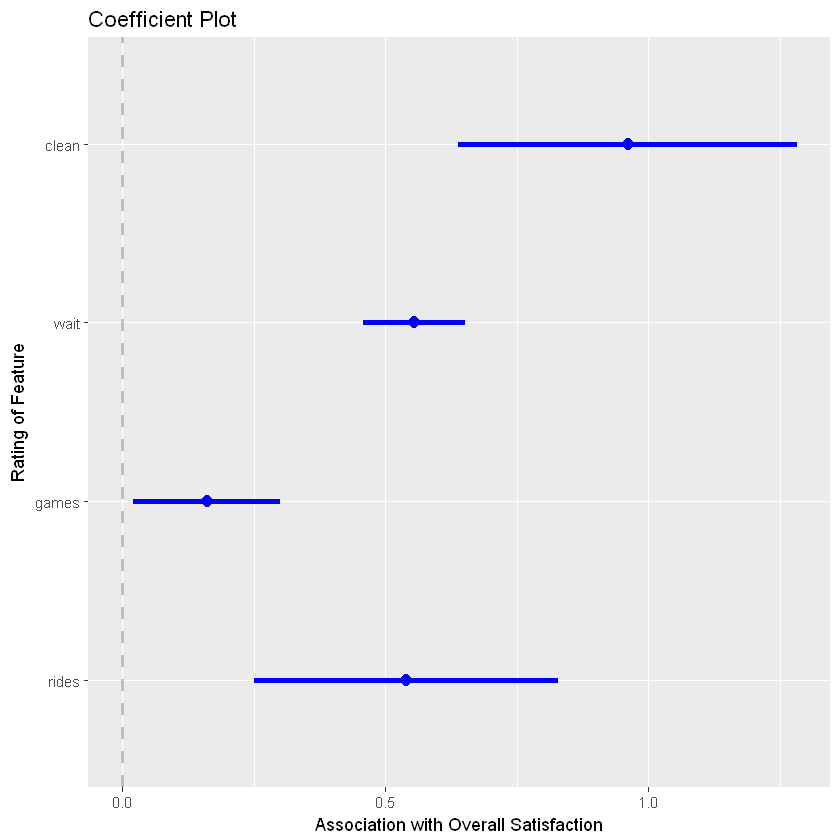We use coefplot() arguments to set the outer confidence interval to a width of 1.96 standard errors (using outerCI=1.96, which corresponds to a 95% confidence interval) and to increase the size of the plotted lines slightly with lwdOuter=1.5. The result is shown in Figure, where we see that satisfaction with cleanliness is estimated to be the most important feature associated with overall satisfaction, followed by satisfaction with the rides and wait times. Satisfaction with games is estimated to be relatively less important. A plot of coefficients is often a key output from a satisfaction drivers analysis. Sorting the plot so that the coefficients are in order based on their estimated coefficient may make it easier to quickly identify the features that are most closely related to overall satisfaction if you have a large number of predictors. Now that we have two model objects, m1 and m2 we might ask which one is better. One way to evaluate models is to compare their R-squared values. summary(m1)$adj.r.squared
summary(m2)$adj.r.squared  0.332430505598762 0.538966781918842 and AIC criterion, which is given by: $AIC = -2\log L + k \times edf ,$ where$L$is likelihood function (in our case based on normal distribution) and$edf$is the number of free parameters for regression model. In OLS it is equal number of independent variables. extractAIC(m1,k=2) extractAIC(m2,k=2)  <ol class=list-inline> <li>2</li> <li>2577.28834706684</li> </ol> <ol class=list-inline> <li>5</li> <li>2395.18049016794</li> </ol> To compare the predictions of the models visually, we plot the fitted versus actual values for each: plot(df$overall, fitted(m1), col="red",xlim=c(0,100), ylim=c(0,100), xlab="Actual Overall Satisfaction", ylab="Fitted Overall Satisfaction")
points(df$overall, fitted(m2), col="blue") legend("topleft", legend=c("model 1", "model 2"),col=c("red", "blue"), pch=1)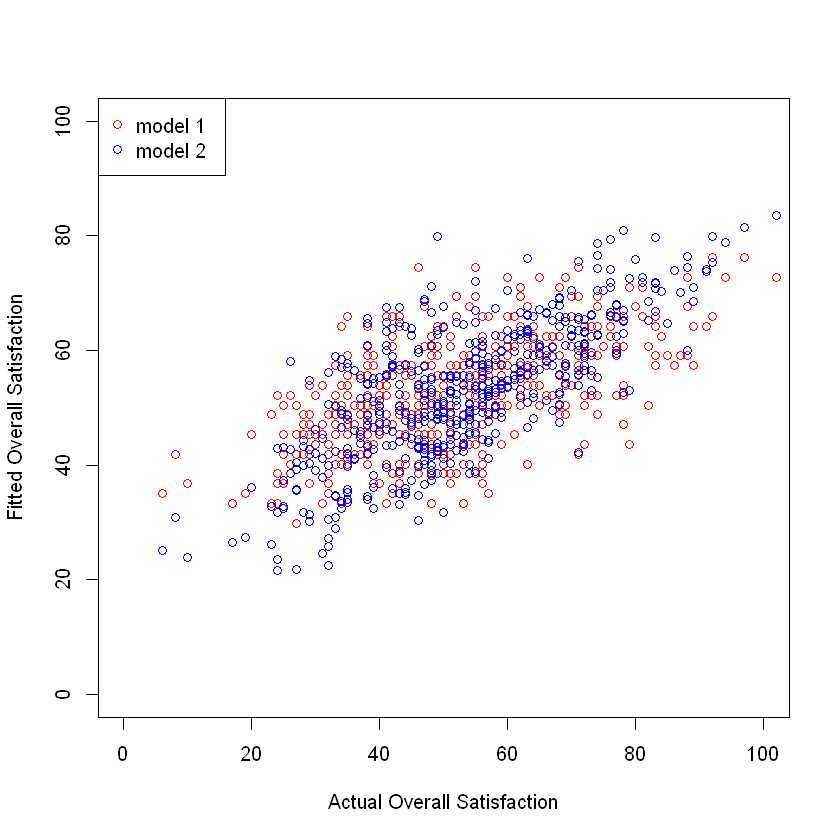If the model fits the data perfectly, it would fall along a 45◦ line in this plot, but, of course, it is nearly impossible to fit customer satisfaction data perfectly. By comparing the red and the blue points in the resulting plot in figure, you can see that the blue cloud of points is more tightly clustered along a diagonal line, which shows that m2 explains more of the variation in the data than m1. # Comparing Nested Models For a more formal test, which is possible because the models here are nested. We can use anova() function to determine whether m2 explains more of the variation than m1: anova(m1, m2)  <th scope=col>Res.Df</th><th scope=col>RSS</th><th scope=col>Df</th><th scope=col>Sum of Sq</th><th scope=col>F</th><th scope=col>Pr(>F)</th> 498 85921.11 NA NA NA NA 495 58980.91 3 26940.2 75.36562 3.641831e-40 The low p-value indicates that the additional predictors in m2 significantly improve the fit of the model. If these two models were the only ones under consideration, we would interpret m2 instead of m1. Thus far, we have interpreted raw coefficients in order to evaluate the contributions of ratings on the shared 100-point scale. However, if the variables have different scales, such as a survey where rides is rated on a 1–10 scale while cleanliness is rated 1–5 scale, then their coefficient values would not be directly comparable. In the present data, this occurs with the distance and logdist variables, which are not on a 100-point scale. When you wish to compare coefficients, it can be helpful to standardize data on a common scale before fitting a model (and after transforming any variables to a more normal scale). The most common standardization converts values to zero-centered units of standard deviation. This subtracts a variable’s mean from each observation and then divides by the standard deviation (sd()). This could be done by: df.std <- df[ , -3] # sat but remove distance df.std[ , 3:8] <- scale(df.std[ , 3:8])  head(df.std)  <th scope=col>weekend</th><th scope=col>num.child</th><th scope=col>rides</th><th scope=col>games</th><th scope=col>wait</th><th scope=col>clean</th><th scope=col>overall</th><th scope=col>logdist</th> yes 0 0.2987971 -0.7561865 -0.87575676 0.1184417 -0.2408509 1.7886823 yes 2 0.2987971 -0.1408004 0.61236008-0.2711692 0.9410106 0.3226360 no 1 -0.2552148 0.1053541 0.05431626 0.1184417 0.5677911 1.1862757 yes 0 0.2987971 -0.7561865 -0.41072025 0.3132472 -0.9250865 0.2803106 no 4 -0.4398855 1.0899720 0.42634547-0.2711692 1.0032138 1.0385034 no 5 -0.8092268 0.1053541 -2.08485169-1.6348075 -1.4849157 0.1452467 ## Model 3 For the next step, we wonder whether satisfaction is different for customers who come on the weekend, travel farther, or have more children.We add these predictors to the model using the standardized data: m3 <- lm(overall ∼ rides + games + wait + clean + weekend + logdist + num.child, data = df.std) summary(m3)  Call: lm(formula = overall ~ rides + games + wait + clean + weekend + logdist + num.child, data = df.std) Residuals: Min 1Q Median 3Q Max -1.60258 -0.39978 0.02281 0.41053 1.70036 Coefficients: Estimate Std. Error t value Pr(>|t|) (Intercept) -0.39447 0.04675 -8.438 3.63e-16 *** rides 0.20641 0.04210 4.903 1.29e-06 *** games 0.07134 0.03041 2.346 0.0194 * wait 0.37871 0.02792 13.566 < 2e-16 *** clean 0.29033 0.04431 6.553 1.43e-10 *** weekendyes -0.04370 0.05167 -0.846 0.3981 logdist 0.06557 0.02585 2.537 0.0115 * num.child 0.23909 0.01720 13.904 < 2e-16 *** --- Signif. codes: 0 '***' 0.001 '**' 0.01 '*' 0.05 '.' 0.1 ' ' 1 Residual standard error: 0.5739 on 492 degrees of freedom Multiple R-squared: 0.6752, Adjusted R-squared: 0.6706 F-statistic: 146.1 on 7 and 492 DF, p-value: < 2.2e-16  When your data includes factors, you must be careful about the data type. For example, num.child is a numeric variable, ranging 0–5, but it doesn’t necessarily make sense to treat it as a number, as we did in m3. In doing so, we implicitly assume that satisfaction goes up or down linearly as a function of the number of children, and that the effect is the same for each additional child. (Anyone who has taken a group of children to an amusement park might guess that this is an unreasonable assumption.) We correct this by converting num.child to a factor and re-estimating the model: df.std$num.child.factor <- factor(df.std$num.child)  m4 <- lm(overall ∼ rides + games + wait + clean + weekend + logdist + num.child.factor, data=df.std) summary(m4)  Call: lm(formula = overall ~ rides + games + wait + clean + weekend + logdist + num.child.factor, data = df.std) Residuals: Min 1Q Median 3Q Max -1.25150 -0.32994 -0.00513 0.31409 1.44218 Coefficients: Estimate Std. Error t value Pr(>|t|) (Intercept) -0.72670 0.04437 -16.378 < 2e-16 *** rides 0.22188 0.03496 6.347 5.01e-10 *** games 0.04299 0.02521 1.705 0.0888 . wait 0.38241 0.02312 16.537 < 2e-16 *** clean 0.29755 0.03675 8.097 4.52e-15 *** weekendyes -0.01880 0.04273 -0.440 0.6602 logdist 0.03154 0.02148 1.468 0.1426 num.child.factor1 1.06124 0.07049 15.056 < 2e-16 *** num.child.factor2 1.08519 0.05575 19.465 < 2e-16 *** num.child.factor3 1.03091 0.06946 14.841 < 2e-16 *** num.child.factor4 0.98893 0.07943 12.450 < 2e-16 *** num.child.factor5 1.04293 0.10253 10.172 < 2e-16 *** --- Signif. codes: 0 '***' 0.001 '**' 0.01 '*' 0.05 '.' 0.1 ' ' 1 Residual standard error: 0.4742 on 488 degrees of freedom Multiple R-squared: 0.7801, Adjusted R-squared: 0.7751 F-statistic: 157.4 on 11 and 488 DF, p-value: < 2.2e-16  A striking thing about m4 is that the increase in overall satisfaction is about the same regardless of how many children there are in the party—about one standard deviation higher for any number of children. This suggests that we don’t actually need to estimate a different increase for each number of children. In fact, if the increase is the same for one child as for five children, attempting to fit a model that scales increasingly per child would result in a less accurate estimate. Instead, we declare a new variable called has.child that is TRUE when the party has children in it and FALSE when the party does not have children. We then estimate the model using that new factor variable. We also drop weekend from the model because it doesn’t seem to be a significant predictor: df.std$has.child <- factor(df.std$num.child > 0) m5 <- lm(overall ∼ rides + games + wait + clean + logdist + has.child,data=df.std) summary(m5)  Call: lm(formula = overall ~ rides + games + wait + clean + logdist + has.child, data = df.std) Residuals: Min 1Q Median 3Q Max -1.26183 -0.33269 -0.00197 0.32233 1.43605 Coefficients: Estimate Std. Error t value Pr(>|t|) (Intercept) -0.73590 0.03861 -19.058 < 2e-16 *** rides 0.22082 0.03466 6.370 4.33e-10 *** games 0.04424 0.02509 1.763 0.0785 . wait 0.38335 0.02299 16.674 < 2e-16 *** clean 0.29785 0.03648 8.164 2.73e-15 *** logdist 0.03443 0.02124 1.621 0.1056 has.childTRUE 1.05429 0.04629 22.774 < 2e-16 *** --- Signif. codes: 0 '***' 0.001 '**' 0.01 '*' 0.05 '.' 0.1 ' ' 1 Residual standard error: 0.4727 on 493 degrees of freedom Multiple R-squared: 0.7792, Adjusted R-squared: 0.7765 F-statistic: 290 on 6 and 493 DF, p-value: < 2.2e-16  We can include an interaction of two terms by using the *: **operator between variables in a formula. For instance, to estimate overall as a function of rides plus the interaction of wait and has.child, we could write the formula as overall *∼ rides + wait:no.child . m6 <- lm(overall ∼ rides + games + wait + clean + weekend + logdist + has.child + rides:has.child + games:has.child + wait:has.child + clean:has.child + rides:weekend + games:weekend + wait:weekend + clean:weekend, data=df.std)  summary(m6)  Call: lm(formula = overall ~ rides + games + wait + clean + weekend + logdist + has.child + rides:has.child + games:has.child + wait:has.child + clean:has.child + rides:weekend + games:weekend + wait:weekend + clean:weekend, data = df.std) Residuals: Min 1Q Median 3Q Max -1.20612 -0.29683 0.00697 0.30788 1.45325 Coefficients: Estimate Std. Error t value Pr(>|t|) (Intercept) -0.714619 0.042563 -16.790 < 2e-16 *** rides 0.146551 0.067344 2.176 0.030026 * games 0.073642 0.048821 1.508 0.132103 wait 0.132772 0.043923 3.023 0.002637 ** clean 0.304566 0.078835 3.863 0.000127 *** weekendyes -0.015968 0.040826 -0.391 0.695880 logdist 0.026443 0.020480 1.291 0.197261 has.childTRUE 1.044456 0.044397 23.525 < 2e-16 *** rides:has.childTRUE 0.054988 0.072208 0.762 0.446719 games:has.childTRUE -0.057629 0.052324 -1.101 0.271275 wait:has.childTRUE 0.341793 0.046910 7.286 1.31e-12 *** clean:has.childTRUE 0.001811 0.078638 0.023 0.981640 rides:weekendyes 0.072338 0.066973 1.080 0.280637 games:weekendyes 0.021666 0.048577 0.446 0.655779 wait:weekendyes 0.033464 0.044053 0.760 0.447846 clean:weekendyes -0.043026 0.070224 -0.613 0.540366 --- Signif. codes: 0 '***' 0.001 '**' 0.01 '*' 0.05 '.' 0.1 ' ' 1 Residual standard error: 0.4477 on 484 degrees of freedom Multiple R-squared: 0.8056, Adjusted R-squared: 0.7996 F-statistic: 133.7 on 15 and 484 DF, p-value: < 2.2e-16  The model object m6 now includes eight interaction terms between ratings for features of the park and no.child and weekend. Only one of these interactions is significant: the wait:no.child interaction. This suggests we could drop the non-significant interactions to create a new model m7: m7 <- lm(overall ∼ rides + games + wait + clean + logdist + has.child + wait:has.child, data=df.std) summary(m7)  Call: lm(formula = overall ~ rides + games + wait + clean + logdist + has.child + wait:has.child, data = df.std) Residuals: Min 1Q Median 3Q Max -1.18731 -0.30473 -0.00076 0.32601 1.41957 Coefficients: Estimate Std. Error t value Pr(>|t|) (Intercept) -0.72769 0.03647 -19.952 < 2e-16 *** rides 0.21439 0.03274 6.549 1.46e-10 *** games 0.04875 0.02370 2.057 0.0402 * wait 0.15182 0.03674 4.133 4.21e-05 *** clean 0.28936 0.03446 8.396 4.96e-16 *** logdist 0.02890 0.02006 1.440 0.1504 has.childTRUE 1.04753 0.04372 23.962 < 2e-16 *** wait:has.childTRUE 0.33970 0.04348 7.813 3.42e-14 *** --- Signif. codes: 0 '***' 0.001 '**' 0.01 '*' 0.05 '.' 0.1 ' ' 1 Residual standard error: 0.4463 on 492 degrees of freedom Multiple R-squared: 0.8036, Adjusted R-squared: 0.8008 F-statistic: 287.6 on 7 and 492 DF, p-value: < 2.2e-16  In these results, we see that attending the park with children is a predictor of higher satisfaction, and waiting time is more important predictor among those with children (wait:has.childTRUE) than those without children. We don’t know the reason for this, but perhaps children go on more rides and their parents are therefore more influenced by wait times. One might further tune the model by considering whether logdist is still needed; I’ll leave that you to verify that and assume that model m7 is the final model. ### What to do with results? What do we do with these results as marketers? We identify several possible marketing interventions. 1. If we want to increase satisfaction overall, we could perhaps do so by trying to increase the number of visitors with children. 2. Alternatively, if we want to appeal to visitors without children, we might engage in further research to understand why their ratings are lower. 3. If we are allocating budget to personnel, the importance of cleanliness suggests continuing to allocate resources there (as opposed, say, to games). 4. We might also want to learn more about the association between children and waiting time, and whether there are things we could do to make waiting less frequent or more enjoyable. There are many more such questions one could pose from results like these; a crucial step in analysis is to think carefully about the implications and where one might be able to make a product or market intervention. Important to remember that the model assesses association, not causation. Possible changes in outcome should be viewed as hypotheses suggested by the model, to be confirmed separately. coefplot(m7, intercept=FALSE, outerCI=1.96, lwdOuter=1.5,ylab="Rating of Feature",xlab="Association with Overall Satisfaction")  Warning message: "Ignoring unknown aesthetics: xmin, xmax"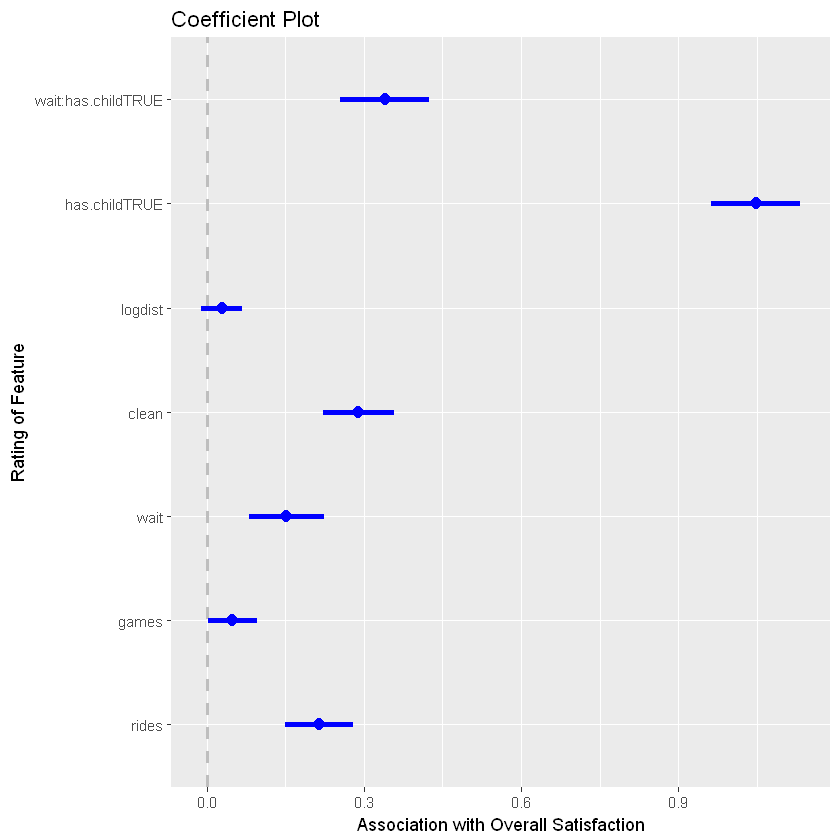# Marginal Plot Marginal Plots provide cool way to validate the functional form of the features. mmps(m7,layout=c(2,3))  Warning message in mmps(m7, layout = c(2, 3)): "Interactions and/or factors skipped"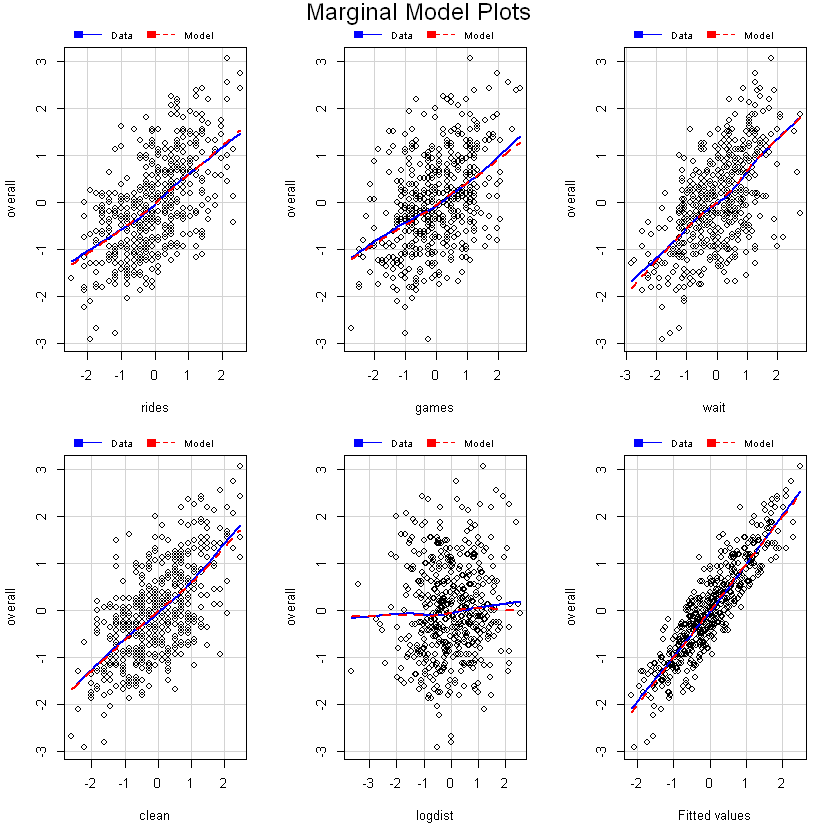# Inverse Response Plot par(mfrow=c(1,1)) inverse.response.plot(m1,key=TRUE)  Warning message: "'inverse.response.plot' is deprecated. Use 'inverseResponsePlot' instead. See help("Deprecated") and help("alr3-deprecated")."  <th scope=col>lambda</th><th scope=col>RSS</th> 1.03740428676.85 -1.00000035249.13 0.00000029794.01 1.00000028677.74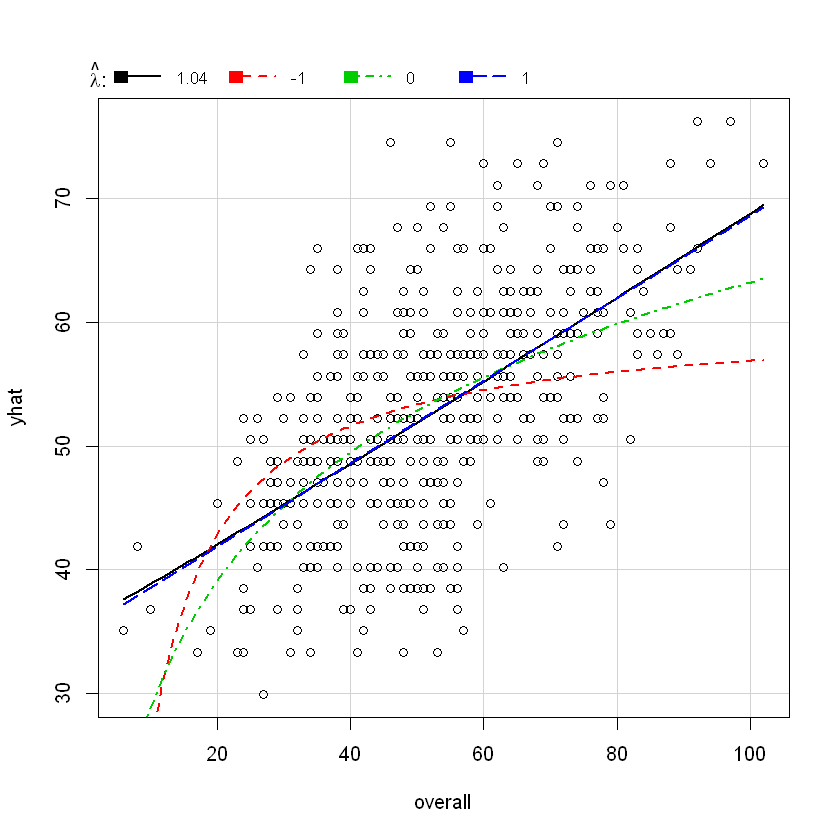#Model=1 n <- length(m1$residuals)
npar <- length(m1$coefficients) +1 #Calculate adjusted R_Square R_sq_m1<-summary(m1)$adj.r.squared
#Calculate AICc
AIC_m1<-extractAIC(m1,k=2)+2*npar*(npar+1)/(n-npar-1)
#Calculate BIC
BIC_m1<-extractAIC(m1,k=log(n))
#Model=2
npar <- length(m2$coefficients) +1 #Calculate adjusted R_Square R_sq_m2<-summary(m2)$adj.r.squared
#Calculate AICc
AIC_m2<-extractAIC(m2,k=2)+2*npar*(npar+1)/(n-npar-1)
#Calculate BIC
BIC_m2<-extractAIC(m2,k=log(n))
#Model=5
npar <- length(m5$coefficients) +1 #Calculate adjusted R_Square R_sq_m5<-summary(m5)$adj.r.squared
#Calculate AICc
AIC_m5<-extractAIC(m5,k=2)+2*npar*(npar+1)/(n-npar-1)
#Calculate BIC
BIC_m5<-extractAIC(m5,k=log(n))
#Model=6
npar <- length(m6$coefficients) +1 #Calculate adjusted R_Square R_sq_m6<-summary(m6)$adj.r.squared
#Calculate AICc
AIC_m6<-extractAIC(m6,k=2)+2*npar*(npar+1)/(n-npar-1)
#Calculate BIC
BIC_m6<-extractAIC(m6,k=log(n))
#Model=6
npar <- length(m7$coefficients) +1 #Calculate adjusted R_Square R_sq_m7<-summary(m7)$adj.r.squared
#Calculate AICc
AIC_m7<-extractAIC(m7,k=2)+2*npar*(npar+1)/(n-npar-1)
#Calculate BIC
BIC_m7<-extractAIC(m7,k=log(n))

R_square<-cbind(R_sq_m1,R_sq_m2,R_sq_m5,R_sq_m6,R_sq_m7)
AIC<-cbind(AIC_m1,AIC_m2,AIC_m5,AIC_m6,AIC_m7)
BIC<-cbind(BIC_m1,BIC_m2,BIC_m5,BIC_m6,BIC_m7)
table<-rbind(R_square,AIC,BIC)


table

<th scope=col>R_sq_m1</th><th scope=col>R_sq_m2</th><th scope=col>R_sq_m5</th><th scope=col>R_sq_m6</th><th scope=col>R_sq_m7</th>
0.3324305 0.5389668 0.7765439 0.799596 0.8008016
2577.33673422395.3508756-742.0264129-786.701850 -798.4242080
2585.71756332416.2535307-712.8174352-720.537830 -765.0746901

## Measuring the quality of fit

Suppose now you want to choose the model based on the measure how good is your model predicting. In the regression setting, the most commonly-use measure is the mean squared error(MSE), given by $MSE = \frac{1}{n}\sum_{i=1}^n(y_i - \hat f(x_i))^2,$ where $\hat f(x_i)$ is the prediction that $hat f$ gives for the $i$th observation. The MSE will be small if the predicted responses are very close to the true responses and vice versa.

Important If you compute estimate to previously seen data set (training data), then it should be comparably small. But in general, we do not really care how well the method works on the training data. Rather, we are interested in the accuracy of the predictions that we obtain when we apply out method to previously unseen test data. When your dataset is small and you do not have luxury to divide your data into two parts. One of the recommended method is $K-$fold crossvalidation

### Cross Validation

In this case we randomly divide the set of observations into $k$ groups, or folds, of approximately equal size. Tje first fold is treated as a validation set, and the method is fit on the remaining $k-1$ folds. The $MSE_1$ is them computed on the observations in the held-out fold. This procedure is repeated $k$ times; each time, a different group of observations is treated as a validation set. This process results in $k$ estimates of the error, $MSE_1,MSE_2,\dots,MSE_k$. The $k-$fold CV is computed by averaging values, $CV_{(k)}=\frac{1}{k}\sum_{i=1}^k MSE_i$

dim(df)
MSE_m5<-deviance(m5)/nobs(m5)
print(MSE_m5)
MSE_m6<-deviance(m6)/nobs(m6)
print(MSE_m6)
MSE_m7<-deviance(m7)/nobs(m7)
print(MSE_m7)


<ol class=list-inline> <li>500</li> <li>9</li> </ol>

 0.2203277
 0.1939911
 0.1960113

cvlm<-function(n_folds=5,model,df)
{
data<-df
K<-n_folds
model<-model
form<-formula(model)
formtxt <- deparse(form)
mf <- model.frame(form, data = data)
ynam <- attr(mf, "names")[attr(attr(mf, "terms"), "response")]
n_fold<-5
sum.sq<-numeric(n_fold)
fold_i <-rep(1:n_fold,length.out=dim(data))
for ( i in 1:n_fold)
{
test_i <-which(fold_i==i)
n<-length(test_i)
test<-data[test_i,]
train<-data[-test_i,]
test_x<-mf[test_i,]
model_i<-lm(form,data=train)
sum.sq[i]<-sum((test$overall-predict(model_i,newdata=test_x))^2)/n } SSE<-sum.sq MSE<-sum(sum.sq)/K return<-list("MSE"=MSE,"SSE"=SSE) return(return) }  MSE.m5<-cvlm(5,m5,df.std)$MSE
MSE.m6<-cvlm(5,m6,df.std)$MSE MSE.m7<-cvlm(5,m7,df.std)$MSE
c(MSE.m5,MSE.m6,MSE.m7)


<ol class=list-inline> <li>0.226783083734997</li> <li>0.207219794257526</li> <li>0.202444613178952</li> </ol>

SSE.m5<-cvlm(5,m5,df.std)$SSE SSE.m6<-cvlm(5,m6,df.std)$SSE
SSE.m7<-cvlm(5,m7,df.std)\$SSE

boxplot(SSE.m5,SSE.m6,SSE.m7,names=c("M5","M6","M7"),col=c("blue","green","purple"))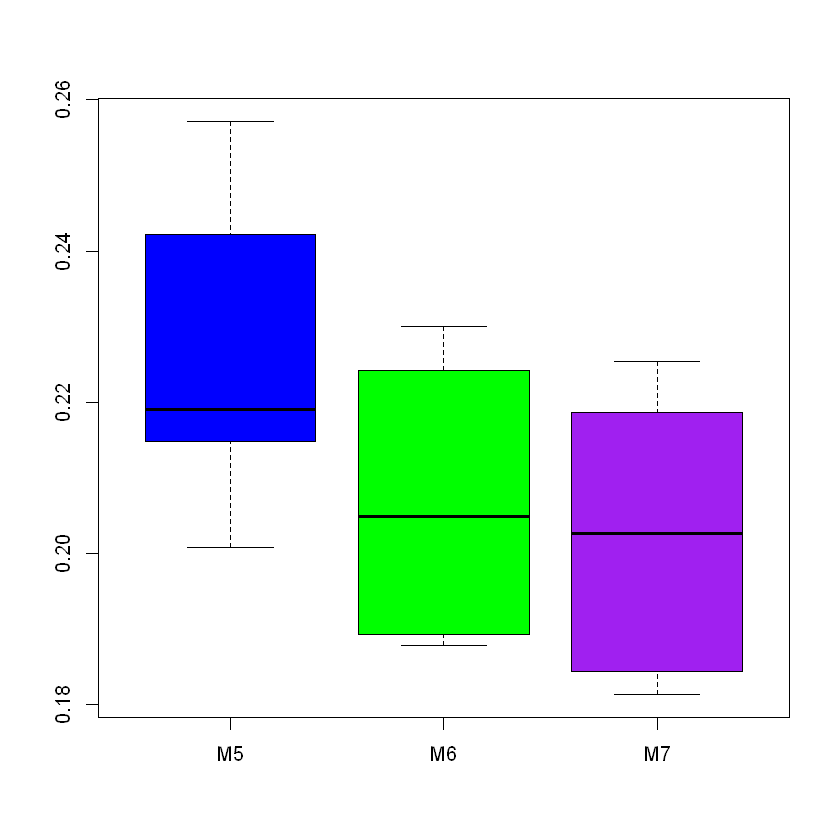We followed a lengthy process to arrive at the final model m7, and it is helpful to recount the general steps we recommend in creating such a linear model. 1. Inspect the data to make sure it is clean and has the structure you expect. 2. Check the distributions of the variables to make sure they are not highly skewed If one is skewed, consider transforming it. 3. Examine the bivariate scatterplots and correlation matrix to see whether there are any extremely correlated variables (such as r > 0.9, or several with r > 0.8). If so, omit some variables or consider transforming them if needed. 4. If you wish to estimate coefficients on a consistent scale, standardize the data with scale() (Sect. 7.3.3). 5. After fitting a model, check the residual quantiles in the output. The residuals show how well the model accounts for the individual observations (Sect. 7.2.4). 6. Check the standard model plots using plot(), which will help you judge whether a linear model is appropriate or whether there is nonlinearity, and will identify potential outliers in the data (Sect. 7.2.4). 7. Try several models and compare them for overall interpretability and model fit by inspecting the residuals’ spread and overall R2 (Sect. 7.3.1). If the models are nested, you could also use anova() for comparison (Sect. 6.5.1) . 8. Report the confidence intervals of the estimates with your interpretation and recommendations (Sect. 7.3).